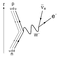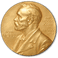# The quark model

01/10/1964

The quark model is a classification scheme for hadrons in terms of their valence quarks—the quarks and antiquarks which give rise to the quantum numbers of the hadrons. The quark model underlies "flavor SU(3)", or the Eightfold Way, the successful classification scheme organizing the large number of lighter hadrons that were being discovered starting in the 1950s and continuing through the 1960s. It received experimental verification beginning in the late 1960s and is a valid effective classification of them to date. The quark model was independently proposed by physicists Murray Gell-Mann, and George Zweig in 1964. Today, the model has essentially been absorbed as a component of the established quantum field theory of strong and electroweak particle interactions, dubbed the Standard Model.

Hadrons are not really "elementary", and can be regarded as bound states of their "valence quarks" and antiquarks, which give rise to the quantum numbers of the hadrons. These quantum numbers are labels identifying the hadrons, and are of two kinds. One set comes from the Poincaré symmetry—JPC, where J, P and C stand for the total angular momentum, P-symmetry, and C-symmetry, respectively.

The remaining are flavor quantum numbers such as the isospin, strangeness, charm, and so on. The strong interactions binding the quarks together are insensitive to these quantum numbers, so variation of them leads to systematic mass and coupling relationships among the hadrons in the same flavor multiplet.

All quarks are assigned a baryon number of ⅓. Up, charm and top quarks have an electric charge of +⅔, while the down, strange, and bottom quarks have an electric charge of −⅓. Antiquarks have the opposite quantum numbers. Quarks are spin-½ particles, and thus fermions. Each quark or antiquark obeys the Gell-Mann−Nishijima formula individually, so any additive assembly of them will as well.

Mesons are made of a valence quark−antiquark pair (thus have a baryon number of 0), while baryons are made of three quarks (thus have a baryon number of 1). This article discusses the quark model for the up, down, and strange flavors of quark (which form an approximate flavor SU(3) symmetry). There are generalizations to larger number of flavors.

#### History

Developing classification schemes for hadrons became a timely question after new experimental techniques uncovered so many of them, that it became clear that they could not all be elementary. These discoveries led Wolfgang Pauli to exclaim "Had I foreseen that, I would have gone into botany." and Enrico Fermi to advise his student Leon Lederman: "Young man, if I could remember the names of these particles, I would have been a botanist." These new schemes earned Nobel prizes for experimental particle physicists, including Luis Alvarez, who was at the forefront of many of these developments. Constructing hadrons as bound states of fewer constituents would thus organize the "zoo" at hand. Several early proposals, such as the ones by Enrico Fermi and Chen-Ning Yang (1949), and the Sakata model (1956), ended up satisfactorily covering the mesons, but failed with baryons, and so were unable to explain all the data.

The Gell-Mann–Nishijima formula, developed by Murray Gell-Mann and Kazuhiko Nishijima, led to the Eightfold way classification, invented by Gell-Mann, with important independent contributions from Yuval Ne'eman, in 1961. The hadrons were organized into SU(3) representation multiplets, octets and decuplets, of roughly the same mass, due to the strong interactions; and smaller mass differences linked to the flavor quantum numbers, invisible to the strong interactions. The Gell-Mann–Okubo mass formula systematized the quantification of these small mass differences among members of a hadronic multiplet, controlled by the explicit symmetry breaking of SU(3).

The spin-3⁄2 Ωbaryon, a member of the ground-state decuplet, was a crucial prediction of that classification. After it was discovered in an experiment at Brookhaven National Laboratory, Gell-Mann received a Nobel prize in physics for his work on the Eightfold Way, in 1969.The pseudoscalar mesonnonet. Members of the octet are shown in green, the singlet in magenta. The na...

Finally, in 1964, Gell-Mann, and, independently, George Zweig, discerned what the Eightfold Way picture encodes. They posited elementary fermionic constituents, unobserved, and possibly unobservable in a free form, underlying and elegantly encoding the Eightfold Way classification, in an economical, tight structure, resulting in further simplicity. Hadronic mass differences were now linked to the different masses of the constituent quarks.

It would take about a decade for the unexpected nature—and physical reality—of these quarks to be appreciated more fully (See Quarks). Counter-intuitively, they cannot ever be observed in isolation (color confinement), but instead always combine with other quarks to form full hadrons, which then furnish ample indirect information on the trapped quarks themselves. Conversely, the quarks serve in the definition of Quantum chromodynamics, the fundamental theory fully describing the strong interactions; and the Eightfold Way is now understood to be a consequence of the flavor symmetry structure of the lightest three of them.

#### Mesons

The Eightfold Way classification is named after the following fact. If we take three flavors of quarks, then the quarks lie in the fundamental representation, 3 (called the triplet) of flavor SU(3). The antiquarks lie in the complex conjugate representation 3. The nine states (nonet) made out of a pair can be decomposed into the trivial representation, 1 (called the singlet), and the adjoint representation, 8 (called the octet). The notation for this decomposition is

The Eightfold Way shows the application of this decomposition to the mesons. If the flavor symmetry were exact (as in the limit that only the strong interactions operate, but the electroweak interactions are notionally switched off), then all nine mesons would have the same mass. However, the physical content of the full theory includes consideration of the symmetry breaking induced by the quark mass differences, and considerations of mixing between various multiplets (such as the octet and the singlet).

Nevertheless, the mass splitting between the η and the η′ is larger than the quark model can accommodate, and this "η – η′ puzzle" has its origin in topological peculiarities of the strong interaction vacuum, such as instanton configurations.

Mesons are hadrons with zero baryon number. If the quark–antiquark pair are in an orbital angular momentum L state, and have spin S, then

• |L − S| ≤ J ≤ L + S, where S = 0 or 1,
• P = (−1)L + 1, where the 1 in the exponent arises from the intrinsic parity of the quark–antiquark pair.
• C = (−1)L + S for mesons which have no flavor. Flavored mesons have indefinite value of C.
• For isospin I = 1 and 0 states, one can define a new multiplicative quantum number called the G-parity such that G = (−1)I + L + S.

If P = (−1)J, then it follows that S = 1, thus PC= 1. States with these quantum numbers are called natural parity states; while all other quantum numbers are thus called exotic (for example the state JPC = 0−−).

#### Baryons

Since quarks are fermions, the spin-statistics theorem implies that the wavefunction of a baryon must be antisymmetric under exchange of any two quarks. This antisymmetric wavefunction is obtained by making it fully antisymmetric in color, discussed below, and symmetric in flavor, spin and space put together. With three flavors, the decomposition in flavor is

The decuplet is symmetric in flavor, the singlet antisymmetric and the two octets have mixed symmetry. The space and spin parts of the states are thereby fixed once the orbital angular momentum is given.

It is sometimes useful to think of the basis states of quarks as the six states of three flavors and two spins per flavor. This approximate symmetry is called spin-flavor SU(6). In terms of this, the decomposition is

The 56 states with symmetric combination of spin and flavour decompose under flavor SU(3) into

where the superscript denotes the spin, S, of the baryon. Since these states are symmetric in spin and flavor, they should also be symmetric in space—a condition that is easily satisfied by making the orbital angular momentum L = 0. These are the ground state baryons.

The S = 1⁄2 octet baryons are the two nucleons (p+ , n0 ), the three Sigmas (Σ+, Σ0, Σ), the two Xis (Ξ0, Ξ ), and the Lambda (Λ0).

The S = 3⁄2 decuplet baryons are the four Deltas ( Δ++, Δ+, Δ0, Δ), three Sigmas ( Σ∗+, Σ∗0, Σ∗−), two Xis (Ξ∗0, Ξ∗−), and the Omega (Ω).

Mixing of baryons, mass splittings within and between multiplets, and magnetic moments are some of the other questions that the model predicts successfully.

#### The discovery of color

Color quantum numbers are the characteristic charges of the strong force, and are completely uninvolved in electroweak interactions. They were discovered as a consequence of the quark model classification, when it was appreciated that the spin S = 3⁄2 baryon, the Δ++, required three up quarks with parallel spins and vanishing orbital angular momentum. Therefore, it could not have an antisymmetric wave function, (due to the Pauli exclusion principle), unless there were a hidden quantum number. Oscar Greenberg noted this problem in 1964, suggesting that quarks should be para-fermions.

Instead, six months later, Moo-Young Han and Yoichiro Nambu suggested the existence of three triplets of quarks to solve this problem, but flavor and color intertwined in that model -- they did not commute.

The modern concept of color completely commuting with all other charges and providing the strong force charge was articulated in 1973, by William Bardeen, Harald Fritzsch, and Murray Gell-Mann.

#### States outside the quark model

While the quark model is derivable from the theory of quantum chromodynamics, the structure of hadrons is more complicated than this model allows. The full quantum mechanicalwave function of any hadron must include virtual quark pairs as well as virtual gluons, and allows for a variety of mixings. There may be hadrons which lie outside the quark model. Among these are the glueballs (which contain only valence gluons), hybrids (which contain valence quarks as well as gluons) and "exotic hadrons" (such as tetraquarks or pentaquarks).

A SCHEMATIC MODEL OF BARYONS AND MESONS - M. GELL- MANN.pdfAN SU3 MODEL FOR STRONG INTERACTION SYMMETRY AND ITS BHEAKING - G. ZWEIG.pdfQuark modelThe Quark Model | A-level Physics | AQA, OCR, EdexcelMurray Gell-Mann on The Quark ModelQuarks | Standard Model Of Particle Physics

REFERENCES

California Institute of Technology. Available in: http://www.caltech.edu/news/50-years-quarks-43351. Access in 11/11/2018.

Wikipedia. Available in: https://en.wikipedia.org/wiki/Quark_model. Access in: 11/11/2018.

Comment

Author

Info

Published in 12/11/2018

Updated in 19/02/2021

All events in the topic Field Theory and Particle Physics:

10/10/1965The Quantum Electrodynamics
28/11/195817/10/1967Electroweak interaction
10/10/1979Glashow, Salam and Weinberg recives the Nobel Prize
01/10/1964The quark model
27/04/1973Asymptotic freedom
10/10/2004D. J. Gross, H. D. Politzer and F. Wilczek receive the Nobel Prize
01/01/197401/01/1977Tau Lepton
10/10/1995M. L. Perl and F. Reines receive the Nobel Prize
15/04/1962Discovery of Muon neutrino
13/07/1983Discovery of carrier particles of weak force W and Z
10/10/1988L. M. Lederman, M. Schwartz and J. Steinberger receive the Nobel Prize
10/10/1984C. Rubbia and S. van der Meer receive the Nobel Prize
04/07/2012The Higgs boson
10/10/2013F. Englert and P. W. Higgs receive the Nobel Prize